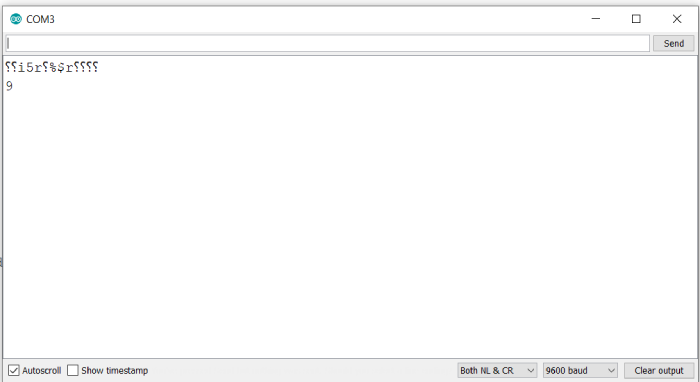# Bitwise XOR in Arduino

Just like other bitwise operators, bitwise XOR also applies on the corresponding bits individually.

The operator is ^ and the syntax is,

a ^ b

where a and b are the two numbers to be XORed.

The truth table for XOR is given below −

PQP^Q
000
011
101
110

As you can see, the XOR operator returns 1 only when the two bits are different.

If you perform 10 ^ 3, this is the computation that will happen at the bit level (assuming your board represents integers using 16 bits)

 0 0 0 0 0 0 0 0 0 0 0 0 1 0 1 0 10 0 0 0 0 0 0 0 0 0 0 0 0 0 0 1 1 3 0 0 0 0 0 0 0 0 0 0 0 0 1 0 0 1 10 ^ 3 = 9

## Example

Let’s verify this on the Serial Monitor. The code is given below −

void setup() {
// put your setup code here, to run once:
Serial.begin(9600);
Serial.println();

int a = 10;
int b = 3;
Serial.println(a ^ b);
}

void loop() {
// put your main code here, to run repeatedly:
}

## Output

The Serial Monitor output is shown below −As you can see, the output is exactly as expected.